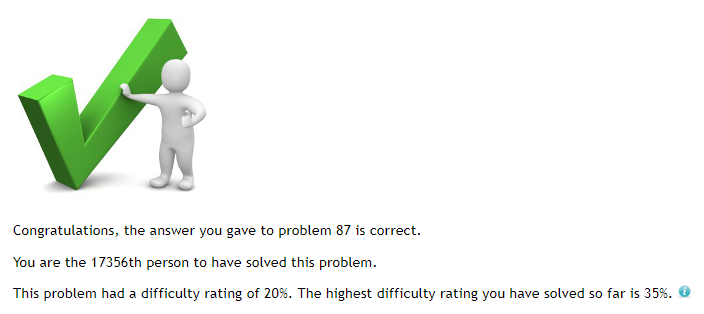# Solving Project Euler Problem 87

Project Euler is a website dedicated to a wide array of complex computational problems. These are my Java solutions to these problems. This blog post reveals my approach to solving the problem, but does not explicitly state the solution. For Project Euler enthusiasts, if you have not completed problem 87 yet, I would recommend not reading this post.

### The problem

The smallest number expressible as the sum of a prime square, prime cube, and prime fourth power is 28. In fact, there are exactly four numbers below fifty that can be expressed in such a way:

• 28 = 22 + 23 + 24
• 33 = 32 + 23 + 24
• 49 = 52 + 23 + 24
• 47 = 22 + 33 + 24

How many numbers below fifty million can be expressed as the sum of a prime square, prime cube, and prime fourth power?

### The greedy algorithm

I last attempted this problem on 10th March - over half a year ago. I decide to discard all of my previous work and start with a fresh new slate. My old code had a triple nested for loop, as well as a never-ending for loop which was clearly some derpy test code.

I come up with a new greedy algorithm design which tries to make the sum by changing the bases of the highest powers. For example, taking 49:

• 49 - 24 = 33
• 49 - 34 = -32

Given that we have gone negative, the algorithm would choose 2 as the base for the 4th power. It would then work out what base to choose for the 3rd and 2nd power using the same method, and backtrack if necessary.

After forming my for loop with a range of 50,000,000, I run the code. And I wait. And wait. And wait… I take a little look at the clock in the corner of my desktop and punch a few numbers into my calculator. I estimate that it would take about 18 minutes for all of this code to run. Oh dear.

On Project Euler’s About page, it specifically states:

Each problem has been designed according to a “one-minute rule”, which means that although it may take several hours to design a successful algorithm with more difficult problems, an efficient implementation will allow a solution to be obtained on a modestly powered computer in less than one minute.

It’s time to revise the algorithm.

### Revising the algorithm

To revise my algorithm, I begin writing comments all over my code, as if I were drawing notes on paper. I quickly find out that I misread the question and forgot that only primes were to be used as the base for each power. At this point, I debate on whether I should fix that issue right here and now, or continue revising the algorithm. I decide to continue revising the algorithm - I’ve spent too long in the past trying to fix issues as they appear and never progressing through a project when I should just brainstorm a bit. Perhaps I didn’t realise that programming isn’t always about writing code - you have to actually think about what you’re writing.

I begin to speculate if there is a way to prove that a number can be expressed as the sum of a prime square, prime cube, and prime fourth power, without actually computing said numbers. At this point, I realise that I should be thinking of forming some sort of constraints on the problem to solve it with less computational power.

I work out (through trial and error) that 50,000,000 - 844 = 212,864, and that 83 in this case is the smallest prime base with power of 4 that does not produce a negative number when subtracted from 50 million. I continue working out the maximum bases for the 3rd and 2nd power, which were 267 and 7069 respectively. At this point, I realise that the “useless code” I wrote 6 months ago was actually useful and that I was really close to a viable solution.

I generate lists of primes up to 7069 using a prime sieve and iterate over them, adding prime square, cube and 4th powers together and storing them in a set. I then use Java’s streams to filter out numbers less than 50 million. Counting the elements in the resultant stream gives the correct solution to the problem, with all of this computation taking less than a second on my computer.And that concludes my solution to Problem 87, which can be viewed (along with all of the notes) on my GitHub repository here.

Written on October 2, 2018